## 5.1.1 Treatment of Moving Boundaries

Chemical and physical processes are used to pattern and to form the surface of microelectronic device structures. The simulation of such surfaces requires on the one hand a rigorous data management to describe the proper material interactions of the reactants and the surface materials as well as on the other hand a huge amount of resources to map the real structure into data structures that can be handled within finite time and computational power.

The first attempt has been introduced decades ago, where the solid is divided into cell structures which also represents solids which can be either added (deposited) or removed (etched). This cellular-based approach has been discussed in [318,172]. However, the simulation of realistic structures is rather limited due to the high demand of memory resources which considerably slows down the simulation if virtual memory has to be assigned for computational purposes.

To overcome this type of limitation, SETIAN and OSHER have introduced a different method to describe moving surfaces and boundaries in [319,320,321,322,323,324]. The level set function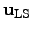describes the evolving surface by a certain speed function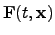as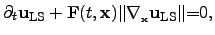(5.1)

where the speed functionincludes the physical effects and chemical reaction like etching, deposition, and diffusion. This speed function has to be specified or modeled for each material type separately. The moving surface is described by the set of points where the level set function is zero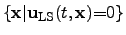(5.2)

and the initial surface is given as the zero points at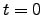: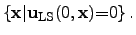(5.3)

The main idea of SETIAN and OSHER was to describe the movement of the three-dimensional surface with a two-dimensional algorithm which decreases the required memory consumption. As a drawback this algorithm requires much less computational power than the cellular-based algorithm. The computational effort to describe a moving surface in three dimensions is for the level-set algorithm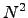compared tofor the cellular-based one. Hence, the level set approach provides a good alternative to expensive computation of evolving surfaces [325,326,327,328,165].

Stefan Holzer 2007-11-19